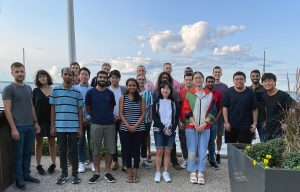# MLOPT Research Group

MLOPT Research GroupThe MLOPT research group is building the mathematical and computational foundations of machine learning and optimization theory. Our work aims to answer fundamental questions about

• the performance limits of machine learning systems
• tradeoffs between data size, computational complexity, and statistical accuracy
• the mathematical characterizations of functions and representations learned from data
• computational and communication complexity in distributed learning and optimization
• privacy and fairness in machine learning and data science
• the interpretability, scalability, and safety of algorithms